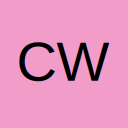# Creating a Density Map via Accumulators, Blobs, and Images

• My incoming image consists of a uniform background and many (thousands) of dark particles. I first find the blobs in the image and have the center of gravity in X and Y of each blob. What I would like to do is then create a "density map" of these blobs as an image output. For instance, if my image is 16000x1000 in size and contains 50,000 blobs, my goal is to create an output image of 160x10 where each 100x100 region of the image is compacted to a single pixel (i.e. image reduces in size by 100x100=10,000x). Each resulting pixel value should then be the sum of the blobs in each 100x100 region of the image. Note that I do not want to count areas of blobs, just the center of gravity of each blob.

For instance, if I have 10 blobs in the first 100x100 pixels of the image, then I want the pixel at (0,0) of my output image to have a value of 10. If there are 56 blobs in the next 100x100 region of the image, then pixel (1,0) of my output image should have a value of 56.

I am new to Visual Applets and it is unclear to me how to do this in an efficient way. I think the design method requires me to first create an image buffer that represents a black image that is 160x10 in size. Then I need to loop through the found blobs CoGs and accumulate the buffer (output) image pixel values depending on where each blob is located. I attached an example picture to this post that shows blobs in a 10x10 image and the CoG of each blob as red dots. Then the red dots are accumulated over each 2x2 region (i.e. 100x100 in example above) and the resulting output image is a 5x5 image count or density map.

Example blob density map.jpg

Any thoughts on the best way to implement this?

• Please use the operator RowSum togehter with ColSum for the basic functionality.

The design below is showing the basic details of the requested algorithm.pasted-from-clipboard.png

Björn Rudde
Field Application Engineer / Consultant - Basler AGCall me on MS Teams

• You can build the COG (X/Y) using Coordinate_X(Y) in the same manner.

Björn Rudde
Field Application Engineer / Consultant - Basler AGCall me on MS Teams

• Bjorn, this is very helpful and I walked through your applet design and it makes sense and is clever. Thank you!

As for using the Coordinate_X(Y) operators, I spent a couple of hours this morning looking through example applets and trying to figure out how to use the COG_X(Y) data to build a 2D image where white pixels are essentially the COG of each detected blob. However, I keep running into issues. I think the problem is mostly to do with the fact that the COG_X(Y) data is a variable stream of 1D data and does not match the image size. For example, my thought was to create a blank image and then create a Coordinate_X and Coordinate_Y image off it. Then to write something like IF(Coordinate_X AND COG_X AND Coordinate_Y AND COG_Y) then 1 else 0. But the Coordinate_X(Y) images are 2D images and the COG_X(Y) data is 1D. Any suggestions on this one?

• ... But the Coordinate_X(Y) images are 2D images and the COG_X(Y) data is 1D. Any suggestions on this one?

Writing all COG X/Y coordinates found by a BlobAnalysis_2D directly into an artificial image is not possible so far. The output of the BlobAnalysis is a list of object features, where the COG's are not sorted by X/Y position. Since these list entries as a result of a of a BlobAnalysis are not sorted it is not possible to reconstruct an image on this basis, because the image is produced linearly: pixel by pixel and line by line, starting at the top left corner.

To solve this it would require a kind of memory. As long as the COG X/Y coordinated describe a small image the operator Histogram can be used. Use CreateBlankImage and SyncToMax to resize the object list to the target resolution and use Y coordinate as MSBits and the X coordinate as LSBits and scale the output into a 2-dimensional image using SplitLine. This will only work in case the required X and Y bits for the ranges are equal or less than 16 bit: That is the maximum number of bits for an histogram.

This would mean that the required resulting image size is 256x256 or less.

Example VA design for that idea ( source RegionCounts_COGxy_BRudde_v02.va ) :

pasted-from-clipboard.png

You can simulate with your image and check the result.

Since the maximum resolution is 256 * 256 pixels in this case, please have a look into the H-Box (norm_COG_XY_List) to see how the area of the oject is used to normalize the COG coordinates and limit these to 8 bit value range:

Björn Rudde
Field Application Engineer / Consultant - Basler AGCall me on MS Teams